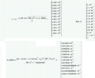# Derivative of a function

• I
1Keenan
Hello,

I would need some help in calculating the derivative of the function T_el in the attached image.
I want to calculate d T_el /d yd, where yd is the variable and it appears in the term I called A_elSide. Its expression is again in the image.
Numbers you see are not important.

Just to explain what I am trying to do:
I want to calculate energy resolution of a magnetic spectrometer (which means distance between two energy point) and it can be calculated as (dT/dy)*spotsize, basically the derivative of the energy with respect to the position on the detector and multiplied by the "nominal" beam spot size.

This means I need to express the energy as a function of the beam position.
Beam position at the detector plane is yd=R*sin(theta)+(d*tg(theta)

First term (R*sin(theta)) is the y coordinate at the magnet output, second term ((d*tg(theta) ) is the additional displacement in the drift d between magnet and detector.
Kinetic energy is in the bending radius R, so doing some math I got an expression for the kinetic enexrgy, which is T_el in the picture

Expression for T_el has an element A_elSide, cotaining the position on the detector (yd), which is the variable with respect I should do the derivative.

An now I need help, I did it already 4 times and I got 4 different results...

Any help?

#### Attachments

•Immagine.png
17.7 KB · Views: 97

Homework Helper
I got 4 different results...
I don't see any of them. Telepathic capabilities severely limited.
And please, ##\LaTeX##, not pictures. Do you want us to do it for you ?

1Keenan
no, I don't want you to do it for me, off course!
I didn't posted them because they are wrong.

My strategy is to derive it as a sqrt((a+f(x))^2) but I guess it doesn't work properly...

Homework Helper
To me it seems the tough part is inverting
yd=R*sin(theta)+(d*tg(theta) )
so I wonder if ##\theta## is small enough to approximate linearly.
(by the way, the thing is called tan, not tg )

My strategy is to derive it as a sqrt((a+f(x))^2)
So what is $${d\over dz} \, \sqrt {a+z^2} \quad ?$$
I didn't posted them because they are wrong.
Post what you think is the best one and solicit comments

1Keenan
Tan or tg is the same...
I'll redo the calculation tomorrow, I think I made a mess with all the other constant terms